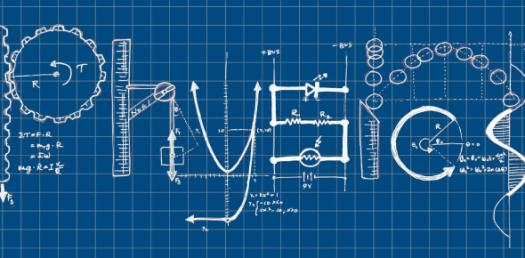# Physics Trivia Quiz On Problem Solving Test!

6 Questions | Total Attempts: 92SettingsAre you looking for a physics trivia quiz on the problem-solving test? You are in luck as the one below is perfect for getting you started on your end of year revision. Do give it a try and be sure to check out the others just like it so as to polish up on your understanding of topics covered. All the best!

• 1.
When an object has a positive velocity and a negative acceleration, what happens to the object's motion?
• A.

The object slows down.

• B.

The object speeds up.

• C.

The object is at rest.

• D.

The object is moving at a constant velocity.

• 2.
A negative acceleration always means an object is slowing down.
• A.

True

• B.

False

• 3.
If the net force on an object is zero, which of the following could be true.  Select all that apply.
• A.

The object is at rest.

• B.

The object is moving at a constant speed to the right.

• C.

The object is moving at a constant speed down.

• D.

The object is getting faster in the x direction.

• 4.
If an object is traveling in a straight line, what is the difference (if any) between the speed and the velocity of the object?
• A.

There is no difference.

• B.

The speed and velocity will be different numbers.

• C.

The velocity and speed must have different units.

• D.

The velocity will have a direction and the speed will not.

• 5.
What is the acceleration (in ) of an object in free fall?  Write only the number (no units) using 2 significant digits.
Related TopicsBack to top